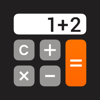Freeware Shareware Font
Home » Top iOS AppsThe Calculator. Impala Studios \$2.99 Category: Utilities Updated: 2010-10-08Description
Get the all-in-one math tool for everyday calculations. Easy functionality & adjustable to your preferences! Join millions of users now and count on The Calculator.

Easy to use and Functional! Start calculating today with the most complete calculator. High productivity is guaranteed due to big buttons and convenient features packed in a visually stunning design.

WHY YOU’LL LOVE THE CALCULATOR
• 4-in1 Calculator;
• Basic, Scientific, Fraction & Currency converter
• History Tape: Save, Copy & Share calculations
• Drag and Drop
• Split View for multitasking support
• Calculate with Degrees or Radians
• Set the number of decimals
• Edit Equation
• Memory Banks
• 70+ Amazing Themes
• Visually impressive & intuitive
• Full Apple Watch support

EDIT EQUATION
You can edit both digits and operators of the current equation.
You can place the edit cursor by tapping or using the arrows.

FRACTIONS
Perform calculations on fractions without converting them to floating point numbers. Floating point numbers that exceed the number of decimal digits are rounded or truncated, generating a relatively small inaccuracy. The solution is to use The Calculator with fractions capabilities.

SHARE YOUR CALCULATION, WHEREVER YOU ARE
Having a great result with your calculation or currency conversion? Save time and send it to your contacts fast & simple… by sharing with The Calculator!

LIST OF MATHEMATICAL OPERATIONS
Cubic Exponent, Cubic Root
Engineering Exponent
Nth Exponent, Nth Root
Power of E, Power of Ten, Power of Two
Square, Square Root
Natural Logarithm, Common Logarithm, Binary Logarithm
Sine, Cosine, Tan
Arc Sine, Arc Cosine, ArcTan
Hyperbolic Sine, Hyperbolic Cosine, Hyperbolic Tan
Hyperbolic Arc Sine, Hyperbolic Arc Cosine, Hyperbolic ArcTan
Multiplicative Inverse, Random Number Input, Factorial

-- WE LOVE FEEDBACK! --
As always, we appreciate your feedback.